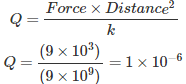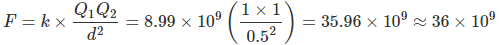# MCQs on Electromagnetic Fields

##### Page 4 of 20. Go to page 1 2 3 4 5 6 7 8 9 10 11 12 13 14 15 16 17 18 19 20
01․ A charge which when placed in vacuum from an equal and similar charge repels with a force of 9 × 103 N, is known as
milli - coulomb.
micro - coulomb.
pico - coulomb.
coulomb.

According to the Coulomb’s law,It is nothing but the micro coulomb.

02․ Voltage applied across a dielectric produces an electrostatic field 50 times greater than air. The dielectric constant of the dielectric will be
50.
10.
20.
5.

Because, the amount of electrostatic field is directly proportional to relative permittivity. This relative permittivity is also called as dielectric constant.

03․ ________ at a point is defined as the force experienced per unit positive charge at a point placed in the electric field.
Electric intensity.
Magnetic flux density.
Electric flux.
None of the above.

Force is experienced by a unit positive charge when placed at a point in an electric field. This force is called as Electric intensity.

04․ The lines of force due to charged particles are
always straight.
always curved.
sometimes curved.
none of above.

The lines of force on charged particles are always curved. The right hand rule can be used to determine the direction of the lines of force.

05․ The ability of charged bodies to exert force on one another is attributed to the existence of
electrons.
protons.
neutrons.
electric field.

Only the movement of electrons can create force on charge. And it would exert force on another nearby charge also.

06․ The electrostatic force between two charges of one coulomb each and placed at a distance of 0.5 m will be
36 × 106 N.
36 × 107 N.
36 × 108 N.
36 × 109 N.07․ Which of the following expression is correct for electric field strength?
E = πD2.
E = πD.
E = D2 / ε.
E = D / ε.

In a dielectric material, the presence of an electric field E causes the bound charges in the material to slightly separate. Hence, the electric displacement field D is defined as D = E × ε.

08․ XEROX machine is working on
electrostatic effect.
magnetic effect.
heating effect of current.
peltier effect.

The process is to spray the electric charge upon the semiconductor surface, and flash the image which would copy and the ink would permanently stick due to the hot pressure from the semiconductor surface.

09․ A point charge in space is attracted towards a dielectric material because of the
phenomenon of dielectric polarisation.
maximisation of electrostatic flux.
dielectric hysteresis.
all of above.

Due to the surface charge density, the particle of a dielectric material carrying the same sign of charge and it is being attracted to one another. Since, the electrostatic flux is directly proportional to the charge density and thus the due to the maximisations of electrostatic flux that point charge is attracted towards a dielectric material.

10․ Coulombs law for the force between electric charges most closely resembles with
Newtons law of motion.
Law of conservation of energy.
Gauss theorem.
Newtons law of gravitation.

Coulomb force is proportional to the product of charges of two objects. Gravitational force is proportional to the product of masses of two objects. Both forces vary inversely as the square of the distance between the two objects, because both laws are inverse square law.

<<<23456>>>